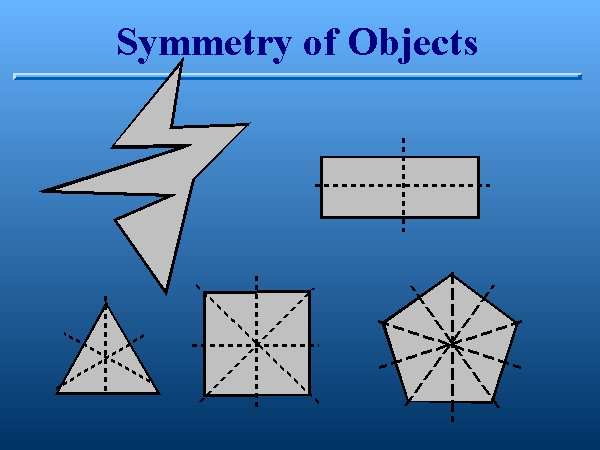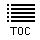Symmetry of Objects
Slide 11 of 24If I asked you which of the following figures is more symmetrical, I think you would intuitively realize that the one in the upper left hand corner is least symmetrical, the rectangle is more symmetrical, the triangle more symmetrical still and so on. This intuitive notion is consistent with both definitions. If you think in terms of balance, the rectangle is balanced about about both lines through the middle of the sides. This notion of balance has an ``operational'' counterpart in the context of Weyl's definition. I can reflect the rectangle through both lines through the center (by flipping it over) and it looks exactly the same. This operation is a symmetry. The triangle clearly has three lines, or axes, about which it is balanced. I can reflect it through any of these three lines and it looks the same. The square has four symmetry axes, a pentagon has five, etc. In this way, the statement that the pentagon is the most symmetrical object on the slide takes on a precise meaning, but one that is consistent with the Oxford dictionary's definition. Hopefully it is also clear that each time you add a side of equal length, you add a symmetry. If you keep the process up ad infinitum you get the most symmetrical two dimensional shape conceivable...